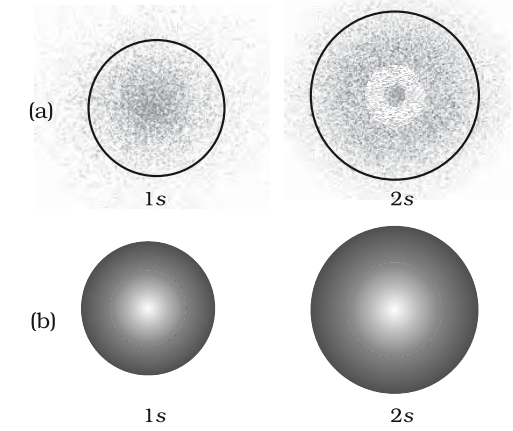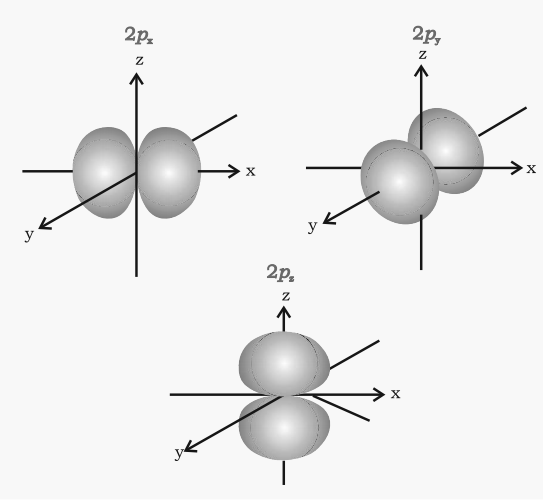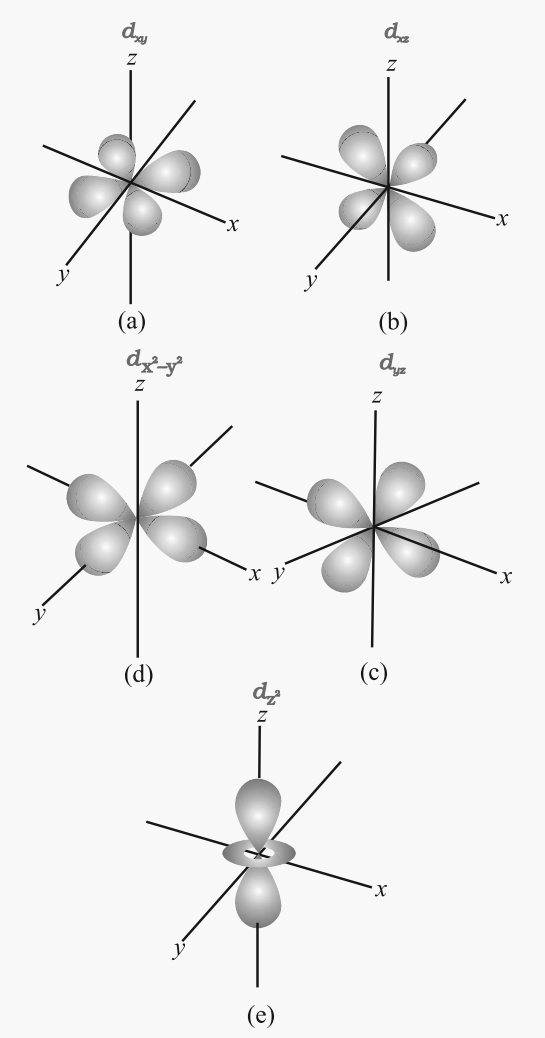# The shape of s-orbitals:

• They are the non-directional and spherically symmetric i.e. probability of finding the electron at a given distance is equal in all directions.
• 1s orbital and 2s orbital have the same shape but size of 2s is larger.
• There is a spherical shell within 2s orbital where the electron density is zero and is called a node.
• The value of azimuthal quantum number(l) is zero (l=0) and magnetic quantum number can have only one value i.e. m = 0.Fig. (a) Probability density plots of 1s and 2s atomic orbitals. The density of the
dots represents the probability density of finding the electron in that region.
(b) Boundary surface diagram for 1s and 2s orbitals

# Shape of p-orbitals

• It consists of two lobes present on either side of the plane that passes through the nucleus. The p-orbital is dumb-bell shaped.
• There are three possible orientations of the electron cloud in p-orbitals. Therefore, the lobes of p-orbital may be considered to be along x,y and z-axis. Hence they are designated as px, py, pz. The three p-orbitals are oriented at right angles to one another.
• First main energy level (Principal quantum number n = 1) does not contain any p-orbital.
• The three p-orbitals of a particular energy level have the same energy in absence of an external electric and magnetic field and are called degenerate orbitals.
• Like s orbitals, p-orbitals increase in size with an increase in the energy of the main shell of an atom. Thus, the value of the azimuthal quantum number is one (l=1) and the magnetic quantum number has three values (m= –1, 0, +1)# Shapes of d-orbitals

• They are designated as dxy, dyz, dzx and dx2-y2. They have a shape like a four-leaf clover. The fifth d orbital designated as dz2 looks like a doughnut.
• All five d orbitals have the same energy in the absence of magnetic field.
• The d orbitals have azimuthal quantum number l = 2 and magnetic quantum number values –2, –1,0,+ 1,+ 2.
• For principal shell number 1 and 2, there are no d orbitals.